# System Downsizing

In this page #1, we would like to explain how Currentier can contribute to "System ( substrate and equipment ) Downsizing".

Current Sensors

With the progress of factory automation in recent years, factories are installing more and more control equipment. Some examples of this control equipment are general-purpose drives for controlling motors, AC servo motor drives for precise position control, and controllers for robots to automate operations. A big challenge for control equipment is to increase the area efficiency of the factory by reducing the size. That is why people have started to consider size reduction of the current detection device and peripheral circuits used for the current control of motors.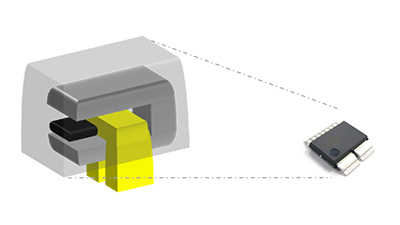Currentier can contribute to system downsizing by three reasons:

1.  low heat,
2.  less peripheral parts and its small package size
3.  speeding up carrier frequency.

## 1. Low Heat

Shunt / cement resistor + isolation ADC / amplifier ( refer to "shunt method" below ) is the mainly used methods for inverter control and vector control. Compared to the shunt method, the total system size of coreless current sensor IC is significantly smaller , not only because the coreless current sensor needs fewer peripheral parts but also because it generates less heat and thus requires less area for heat dissipation.

Shunt method is a method that generates a voltage from a resistor, and the resistance value needs to be large enough to ensure the output voltage. Thus, even the heat-resistance temperature of the resistor can be higher, it is difficult to control heat generation itself in principle.

In contrast, coreless current sensor is a method that converting the magnetic field generated by the measured current flowing through the primary conductor into a voltage using a magnetic sensor, so that there is no need to increase the value of the resistance of the primary conductor.

Especially for Currentier, it can make the resistance lower and suppress the heat generation by making the primary conductor wider. It is because Currentier is capable of effective voltage conversion in relatively weak magnetic fields by using AKM's high sensitive magnetic sensor ( compound semiconductor ).

Figure 1. shows  the heat generation result ( thermal image ) of Currentier ( CZ375 series CZ372, CZ370 series ) and shunt method / a general coreless current sensor.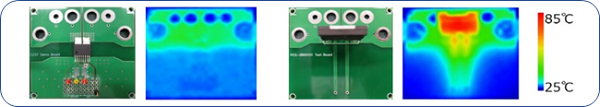Figure 1. The heat generation result comparison of Currentier and shunt resistor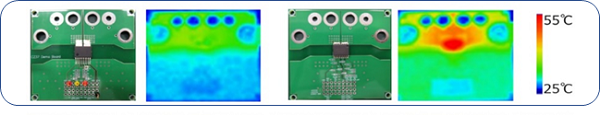Figure 2. The heat generation result comparison of Currentier and general coreless current sensor

It is obvious from the thermal images above that there is a big difference in the heat generation. When 40A is applied for 10 minuets, Currentier generates about 50℃ less heat than a shunt method, and about 20℃ less heat than a general coreless current sensor.

Currentier is lower resistance and lower heat generation compared with the shunt method, so that it can reduce the board area for heat dissipation.

For example, if the rated current is 30Arms and the current range is 85App, the resistance value is approximately 2.4mΩ in the shunt method. (*input voltage of isolation amplifier is 200mV ) In this case, the heat generation is about 2.1W.

But in the case of Currentier ( CZ375, CZ372, CZ370 series ) , its heat generation is about 0.24mW because its resistance value is 0.27mΩ, unchanged by the amount of current.

Assuming the use of two current detections which is common in phase current detection in general inverters,  to reduce this heat generation to a temperature rise of Δ20℃,

Shunt method : 56cm^2 ( ≒7.5cm per one side ) *2 places

CZ375, CZ372, CZ370 series    :  6.4cm^2 ( ≒2.5cm per one side ) *2 places

are required and Currentier can achieve about 90% area reduction.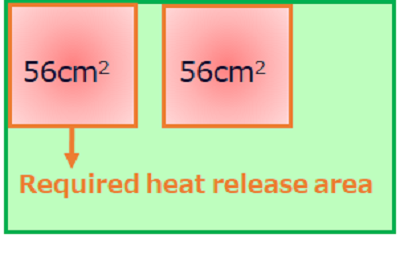Figure 3. Example of using shunt resistor + isolation amplifier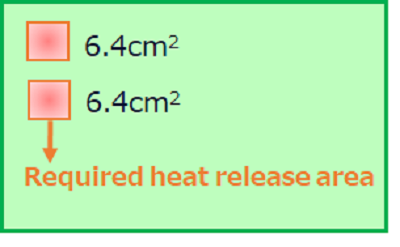Figure 4. Example of using Currentier

## 2. Less Peripheral Parts and Smaller Package Size

Unlike the shunt method, Currentier does not need a complex run of an isolated power supply.
Therefore, the number of peripheral parts can be less and also the board size can be reduced. ( Figure 5 & 6 )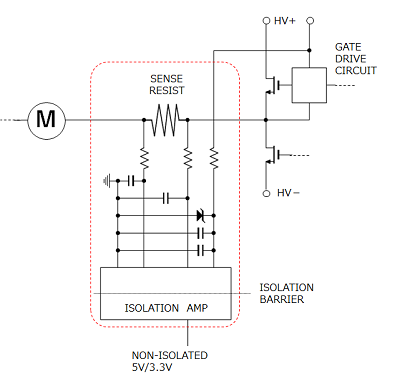Figure 5. Circuit diagram with shunt resistor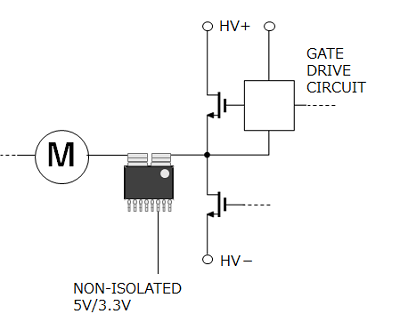Figure 6. Circuit diagram with Currentier

Besides, Currentier itself is so small that the height and mounting area can be significantly reduced compared to a cored current sensor. ( Figure 7 )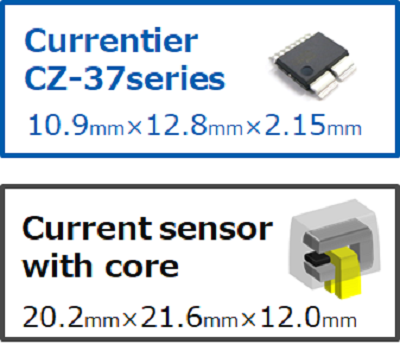Figure 7. Products comparison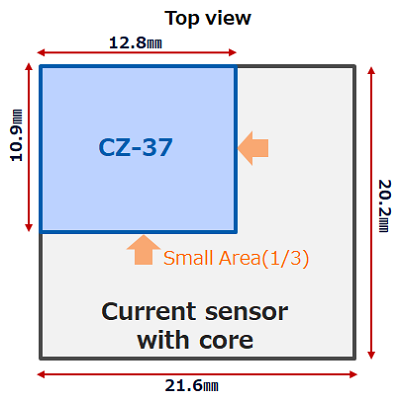Comparison of projected area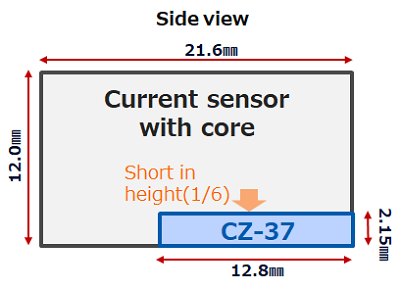Comparison of height

## 3. Speeding up Carrier Frequency

Because Currentier supports high speed frequencies, the size of the transformer, capacitor, and inductor can be reduced in PFC circuits, which can contribute to system downsizing. ( Figure 8 )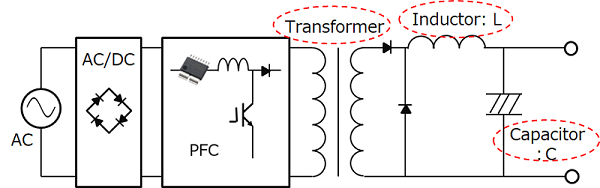Figure 8. Schematic of the power supply unit block diagram

General coreless current sensor uses a low-sensitive Silicon (Si) hall element, so that the application factor needs to be higher enough. If the frequency band is to be wide, the noise would also be increased, so that the frequency band has to be narrowed to counteract the noise. As a result of that, the response time become slow.

In contrast, Currentier uses a high sensitive hall element, thus it can make the application factor lower and the wide bandwidth can be secured. Accordingly, compared to current sensor with Si hall element,  its response time is faster and it can follow high speed frequency. ( Figure 9 )

The transformers, inductors and capacitors used in the PFC circuits of switching power supply have to be enlarged in size in order to be used at low switching frequencies, and these parts take up a large area of the substrate. By using a current sensor that can follow a high frequency to speed up the switching frequency, the transformer is less likely to be magnetically saturated even it is small. Even if the inductor has a small inductance L and the capacitor has a small electric capacity C, sufficient impedance can be obtained. As a result of that, the downsizing of switching power supply can be achieved.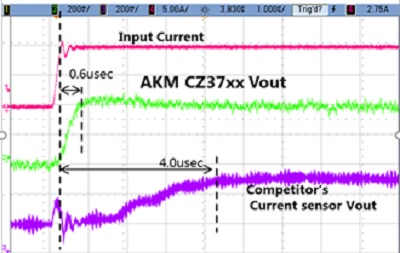Figure 9. Response time comparison of CZ37 and general coreless current sensor IC

## Summary

Currentier can contributes to system downsizing by

•  Downsizing of substrate by low heat
•  Reduction in the number of peripheral parts and downsizing of current sensor itself
•  Downsizing of peripheral parts by speeding up carrier frequency

## Recommend

### Solutions

If you would like to know more about how Currentier can solve problems, please click below links.

### Applications

If you would like to consider proper products from application examples, please click below links.

AC Servo / General Inverter

Photovoltaic Inverter

### Products

For more detailed information about each product series, and for selection of specific products, please click below links.

Lineup

Selection Guide